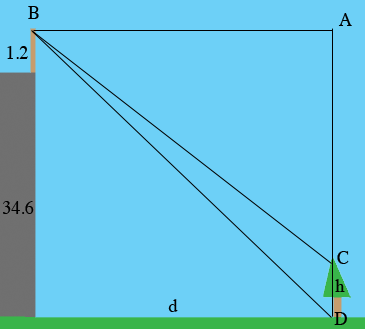SEARCH HOMEMath Central Quandaries & QueriesQuestion from Kerissa: A man height 1.2 m standing on top of a building 34.6m high views a tree some distance away he observes that the angle of depression of the base of the tree is 35 and the angle of depression of the top of the tree is 29, assuming that the tree stands of level ground. Determine the height of the tree.Hi,

I can help get you started.

Here is my diagram. The building is gray, the light brown is the man, the height of the tree is $h$ meters, and the distance from the base of the building to the base of the tree is $d$ meters.The measure of the angle $ABC$ is 29 degrees and the measure of the angle $ABD$ is 35 degrees.

What is $d?$

PennyMath Central is supported by the University of Regina and The Pacific Institute for the Mathematical Sciences.